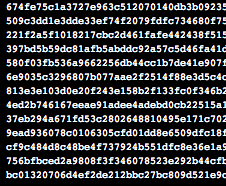# Quantum computing is so powerful it takes two years to understand what happened

## Boffins: 'We factored 143', 'no, you factored 56,153', which is bad news for cryptoIn 2012 a group of Chinese quantum physicists pulled off an acclaimed success in quantum-based factoring, running an adiabatic quantum algorithm for the number 143, at the time believed to be the largest number ever factored in a quantum computation.

It now seems that paper, here, could have overlooked something: in a new paper at Arxiv, Nikesh Dattani and Nathaniel Bryans (Kyoto University and the University of Calgary, respectively, believe that the computation happened to also factor the numbers 3,599, 11,663 and 56,153.

The difference between the two: the original Chinese work happened to factor not just a number (143), but in fact a whole class of numbers of which 143 is a member.

While even 56,153 is a tiny number compared to the kind of factorisation predicted by fans of Shor's algorithm (and feared by spooks), it was accomplished using only four qubits – which means that the work can be reproduced and therefore validated on a classical computer without waiting an unreasonable time to test the work.

Let's start with the original paper. It presents a demonstration that used liquid crystal nuclear magnetic resonance – NMR – as the quantum computer, operating at 300 Kelvin with four qubits represented as nuclear spins.

(From there, Vulture South has to confess being beaten by the mathematics of the first paper, but it concludes that “this is the first realisation of quantum algorithms to factor a number larger than 100”).

The newer authors make this startling claim: since the four-qubit factorisations can be performed on classical computers, they could try to replicate it, and that's where the surprise comes in: 143, the number originally demonstrated, has a kind of “collision” with the other numbers:

“These have precisely the same form as the equations in the factorisation of 143, except with different variables. Therefore the Hamiltonian is also the same, except the qubits … represent different positions in the corresponding binary strings … Other numbers that we have discovered reduce to the same equations include 3,599, 11,663 and 56,153”.

Even better, from the researchers' point of view, is that this represents a considerable jump compared to attempts to implement Shor's algorithm (the first proposed use of a quantum computer for factorisation).

So far, every shot at using a quantum computer to solve Shor's algorithm could only be tested if the researcher already knew the answer, while this minimisation approach has factorised 56,153 without being able to peek in the back of the book.

Which takes the world a considerable distance towards being able to demonstrate the kind of quantum-based factorisation that would eventually obsolete current cryptography. ®